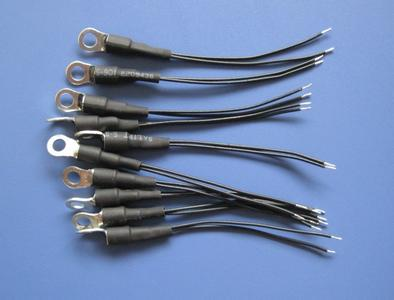﻿ What is the meaning of the linear NTC temperature sensor reference voltage?_

China sensor manufacturers

# What is the meaning of the linear NTC temperature sensor reference voltage?

What is the meaning of the linear NTC temperature sensor reference voltage?
The reference voltage is the voltage value at the sensor's temperature field (ice-water mixture) at 0 °C and the operating current (100 μA). In fact, it is the 0 point voltage. The symbol is V(0), and the value is calibrated at the factory. Since the temperature coefficient S of the sensor is the same, the reference voltage value V(0) is known. The sensor voltage at any temperature point can be ascertained without having to index the sensor. Its calculation formula is:
V(T)=V(0)+S×T
Example: such as reference voltage V (0) = 700 mV; The temperature coefficient S = -2 mV / ° C, then at 50 ° C, the sensor output voltage V (50) = 700 - 2 × 50 = 600 (mV). This is where the linear temperature sensor is superior to other temperature sensors.﻿
﻿